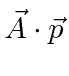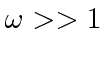## Scattering of Photons

34 The quantized photon field can be used to compute the cross section for photon scattering. The electric dipole approximation is used to simplify the atomic matrix element at low energy where the wavelength is long compared to atomic size.

To scatter a photon the field must act twice, once to annihilate the initial state photon and once to create the final state photon. Since the quantized field contains both creation and annihilation operators,either theterm in first order, or theterm in second order can contribute to scattering. Both of these amplitudes are of order.

The matrix element of theterm to go from a photon of wave vectorand an atomic stateto a scattered photon of wave vectorand an atomic stateis particularly simple since it contains no atomic coordinates or momenta.The second order terms can change atomic states because of theoperator.

The cross section for photon scattering is then given by theKramers-Heisenberg Formula. The three terms come from the three Feynman diagrams that contribute to the scattering to order.

This result can be specialized for the case of elastic scattering, with the help of some commutators.Lord Rayleigh calculated low energy elastic scattering of light from atoms using classical electromagnetism. If the energy of the scattered photon is less than the energy needed to excite the atom, then the cross section is proportional to, so that blue light scatters more than red light does in the colorless gasses in our atmosphere.

If the energy of the scattered photon is much bigger than the binding energy of the atom,eV. then the cross section approaches that for scattering from a free electron, Thomson Scattering.The scattering is roughly energy independent and the only angular dependence is on polarization. Scattered light can be polarized even if incident light is not.

Jim Branson 2013-04-22